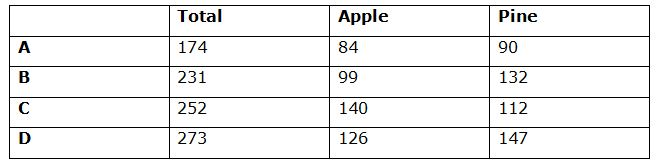# IBPS RRB Clerk/EPFO Assistant Prelims Quantitative Aptitude Questions 2019 (Day-12)

Dear Aspirants, Our IBPS Guide team is providing new series of Quantitative Aptitude Questions for IBPS RRB Clerk/EPFO Assistant Prelims 2019 so the aspirants can practice it on a daily basis. These questions are framed by our skilled experts after understanding your needs thoroughly. Aspirants can practice these new series questions daily to familiarize with the exact exam pattern and make your preparation effective.

### Click Here to Subscribe Crack High Level Puzzles & Seating Arrangement Questions PDF 2019 Plan

[WpProQuiz 6764]

Directions (1 – 5): Study the following information carefully and answer the questions given below.

There are 4 villages A, B, C and D, which have some apple and pine trees. The ratio of apple and pine trees in these villages is 14: 15, 3: 4, 5: 4 and 6: 7 respectively. The difference between the apple and pine trees in village D is 21. The difference between apple trees between the village C and D respectively is 14. The ratio of the apple and pine trees in A and C respectively is 3: 4. The difference between the apple and pine trees in B and A respectively is 9.

1) Find the total number of pine trees in all the villages.

a) 418

b) 814

c) 184

d) 481

e) None of these

2) If 30% of pine trees in village A is planted in village B, then what will be the effect in total number of trees in village B?

a) 25% increase

b) 5% increase

c) 20% increase

d) 22% increase

e) None of these

3) The number of apple trees in D is what percentage more than the number of pine trees in A?

a) 40%

b) 140%

c) 20%

d) 120%

e) None of these

4) Find the difference between the average number of apple trees in A and D and the number of pine trees in B and C.

a) 34

b) 36

c) 17

d) 18

e) None of these

5) If all the trees in B are apple and 20% apple trees of village C is added to the apple trees in D, the apple trees in B is what percentage of number of apple trees in D?

a) 250%

b) 150%

c) 50%

d) 75%

e) None of these

6) A certain sum of money amounts to Rs2710.55 in three years and Rs2828.4 in 4 years in simple interest. Find the rate of interest.

a) 10%

b) 20%

c) 15%

d) 5%

e) None of these

7) Find the compound interest on the sum Rs3600 for 4 years at the rate of 4% per annum compounded annually.

a) Rs549.23

b) Rs754.32

c) Rs456.21

d) Rs265.87

e) None of these

8) The ratio of milk and water in container A is 5: 4 and in B is 5: 7. If this mixture was mixed together the ratio of milk and water in final mixture can be:

a) 35:37

b) 75:37

c) 23:65

e) None of these

9) Pipes A, B and C can fill a tank in 20h. If pipe A and B alone can fill the tank in 80 h and 60 h respectively, find the time taken by C alone to fill the tank.

a) 12 h

b) 24 h

c) 48 h

d) 96 h

e) None of these

10) A bag contains 5 red balls, 6 blue balls and 7 pink balls. Two balls taken at random, find the probability that the balls are in same color.

a) 23/156

b) 46/153

c) 38/153

d) 41/153

e) None of these

Direction (1 – 5):

The ratio of apple and pine trees in village,

A = 14: 15

B =3: 4

C = 5: 4

D = 6: 7

D:

The ratio = 6: 7 (6x, 7x)

Given that, 7x – 6x = 21

x = 21

Apple = 6 * 21 = 126

Pine = 7 * 21 = 147

C:

Apple = 126 + 14 = 140

Pine = (140/5) * 4 = 112

A:

The ratio of the apple and pine trees in A and C respectively = 3: 4

Apple = (112/4) * 3 = 84

Pine = (84/14) * 15 = 90

B:

Apple = 90+ 9 = 99

Pine = (99/3) * 4 = 132Required sum = 90 + 132 + 112 + 147 = 481

Added trees = (30/100) * 90 = 27

Required percentage = (27/231) * 100 = 11.68%

Required percentage = [(126 – 90)/90] * 100 = 40%

Required difference = [(132 + 112)/2]–[(84 + 126)/2] = 17

Apple trees in B = 99 + 132 = 231

Apple trees in D = 126 + 140 * (20/100) = 154

Required percentage = (231/154) * 100 = 150%

Direction (6-10) :

SI = pnr/100

SI for one year = 2828.4 – 2710.55 = Rs117.85

SI for 3 year = 117.85 * 3 = Rs353.55

p = 2710.55 – 353.55 = Rs2357

r = (117.85/2357) * 100 = 5%

Compound interest = p (1 + r/100)n– p

CI = 3600 (1 + 4/100)4–3600

CI = Rs611.49

Milk in A = 5/9

Water in A = 4/9

Milk in B = 5/12

Water in B = 7/12

In final mixture milk: water = (5/9 + 5/12): (4/9 + 7/12) = 35: 37

Pipes fill the tank in one hour,

A + B + C = 1/20

A = 1/80

B = 1/60

C = 1/20 – 1/80 – 1/60

C = 1/48

Pipe C alone can fill the tank in 48 h.